# 5 以 ggplot2 進行資料視覺化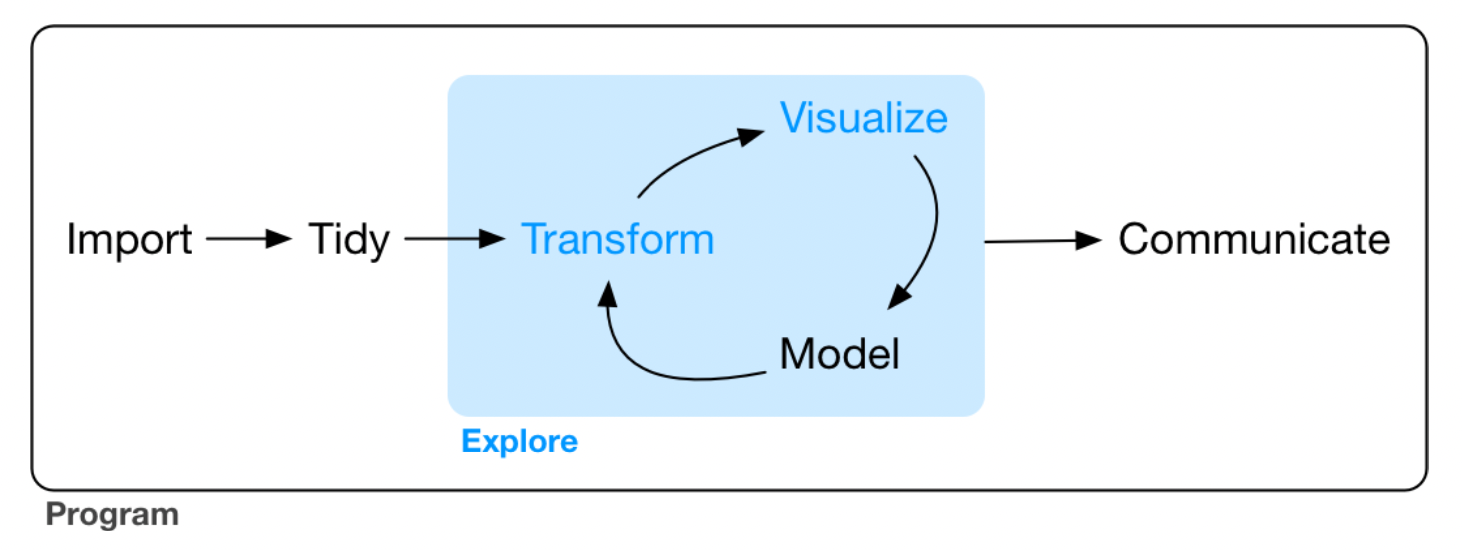Figure 5.1: Data exploring.

### 前置作業

library(tidyverse)
## ── Attaching packages ─────────────────────────────────────── tidyverse 1.3.1 ──
## ✓ ggplot2 3.3.5     ✓ purrr   0.3.4
## ✓ tibble  3.1.3     ✓ dplyr   1.0.7
## ✓ tidyr   1.1.3     ✓ stringr 1.4.0
## ✓ readr   2.0.1     ✓ forcats 0.5.1
## ── Conflicts ────────────────────────────────────────── tidyverse_conflicts() ──
## x purrr::transpose()      masks data.table::transpose()

## 5.1 創建一個 ggplot

mpg
## # A tibble: 234 × 11
##    manufacturer model      displ  year   cyl trans drv     cty   hwy fl    class
##    <chr>        <chr>      <dbl> <int> <int> <chr> <chr> <int> <int> <chr> <chr>
##  1 audi         a4           1.8  1999     4 auto… f        18    29 p     comp…
##  2 audi         a4           1.8  1999     4 manu… f        21    29 p     comp…
##  3 audi         a4           2    2008     4 manu… f        20    31 p     comp…
##  4 audi         a4           2    2008     4 auto… f        21    30 p     comp…
##  5 audi         a4           2.8  1999     6 auto… f        16    26 p     comp…
##  6 audi         a4           2.8  1999     6 manu… f        18    26 p     comp…
##  7 audi         a4           3.1  2008     6 auto… f        18    27 p     comp…
##  8 audi         a4 quattro   1.8  1999     4 manu… 4        18    26 p     comp…
##  9 audi         a4 quattro   1.8  1999     4 auto… 4        16    25 p     comp…
## 10 audi         a4 quattro   2    2008     4 manu… 4        20    28 p     comp…
## # … with 224 more rows

ggplot(data = mpg) + geom_point(mapping = aes(x = displ, y = hwy))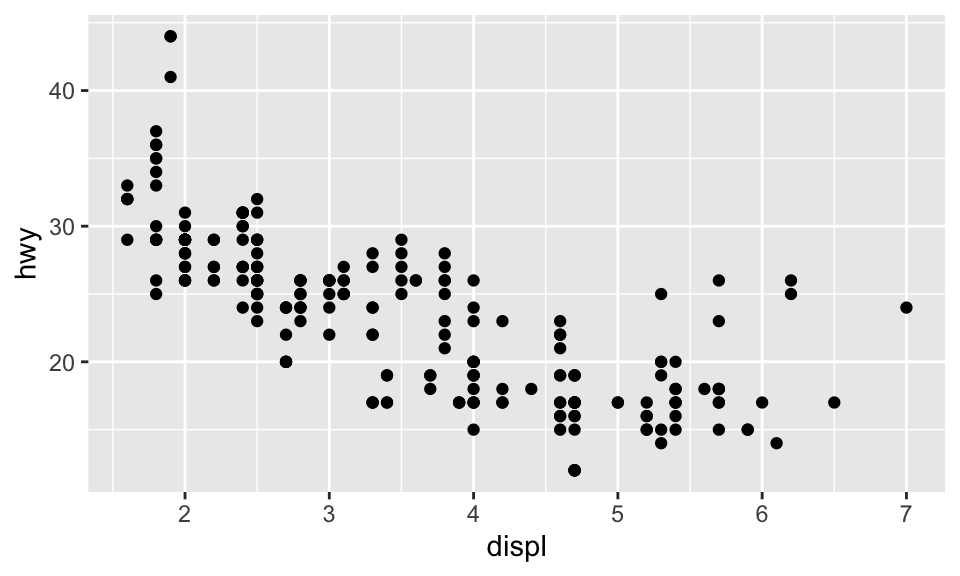ggplot() 可以創造一個座標系統，而我們可以在上面加上圖層。其中，第一個引數為此圖所要使用的 dataset，例如此處為 ggplot(dataset=mpg)，但這時候不會得到任何東西，只有一張空白的圖。而我們可以再加上其他圖層，如使用 geom_point()，可以用來繪製散佈圖（scatterplot）。而 geom_point() 函數有引數 mapping，與 aes() 搭配使用，可以讓我們指定 $$x$$ 軸與 $$y$$ 軸分別要是什麼變數。

## 5.2 The Layered Grammar of Graphics

ggplot2 的語法大致如下，層層堆疊各種函數。在 geom 中，除了 mapping=aes()，我們還可以加上其他種類的 stat 與 “position adjustment”；若有需要，也可以加上不同的「座標系統」與 “facet function”。

ggplot(data = <DATA>) +
<GEOM_FUNCTION>(
mapping = aes(<MAPPINGS>),
stat = <STAT>,
position = <POSITION>)+
<COORDINATE_FUNCTION> +
<FACET_FUNCTION>

## 5.3 Aesthetic Mappings

# ggplot(data = mpg) +
#   geom_point(mapping = aes(x = displ, y = hwy, color = class), show.legend = FALSE)
ggplot(data = mpg) + geom_point(mapping = aes(x = displ, y = hwy, color = class))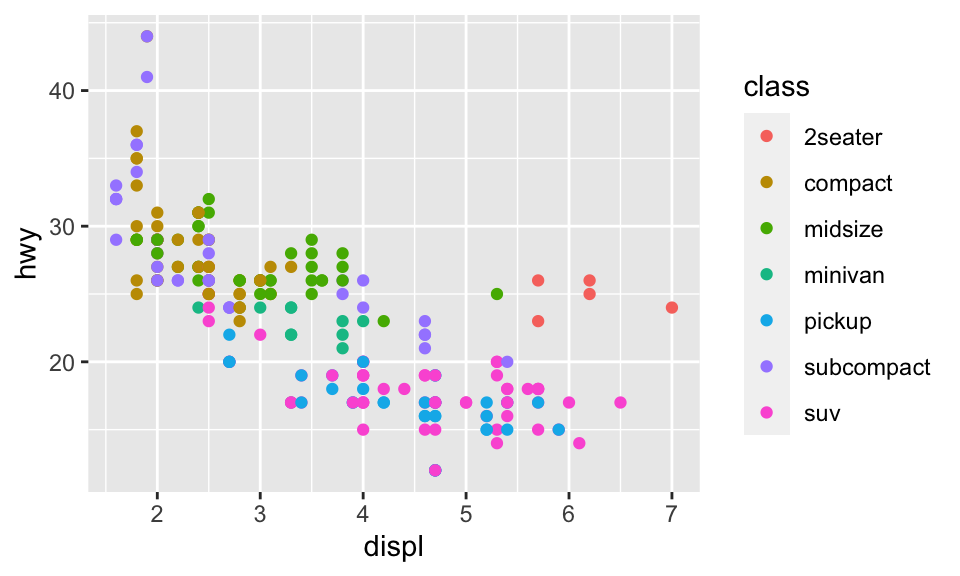ggplot(data = mpg) + geom_point(mapping = aes(x = displ, y = hwy, color = displ < 5))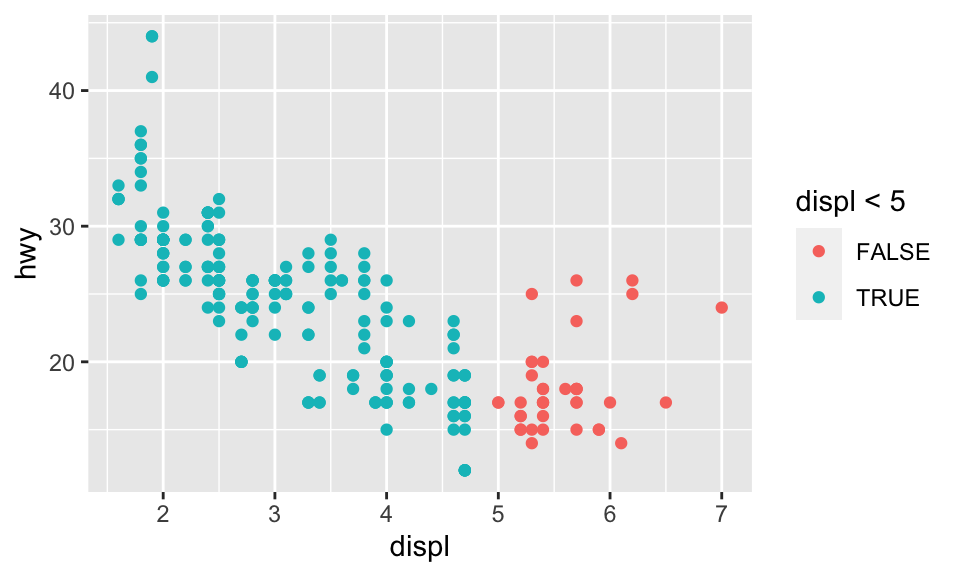ggplot(data = mpg) + geom_point(mapping = aes(x = displ, y = hwy, size = class))
## Warning: Using size for a discrete variable is not advised.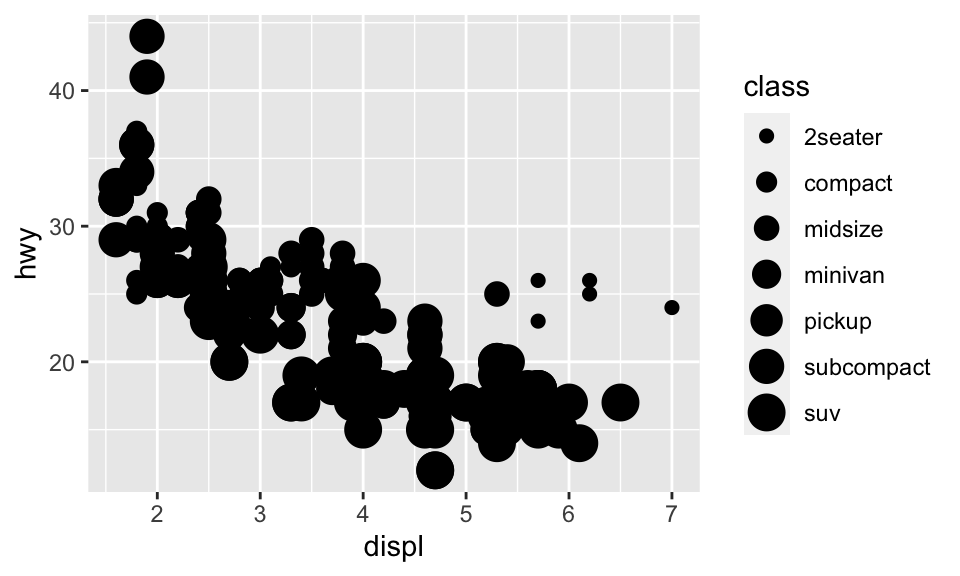ggplot(data = mpg) + geom_point(mapping = aes(x = displ, y = hwy, alpha = class))
## Warning: Using alpha for a discrete variable is not advised.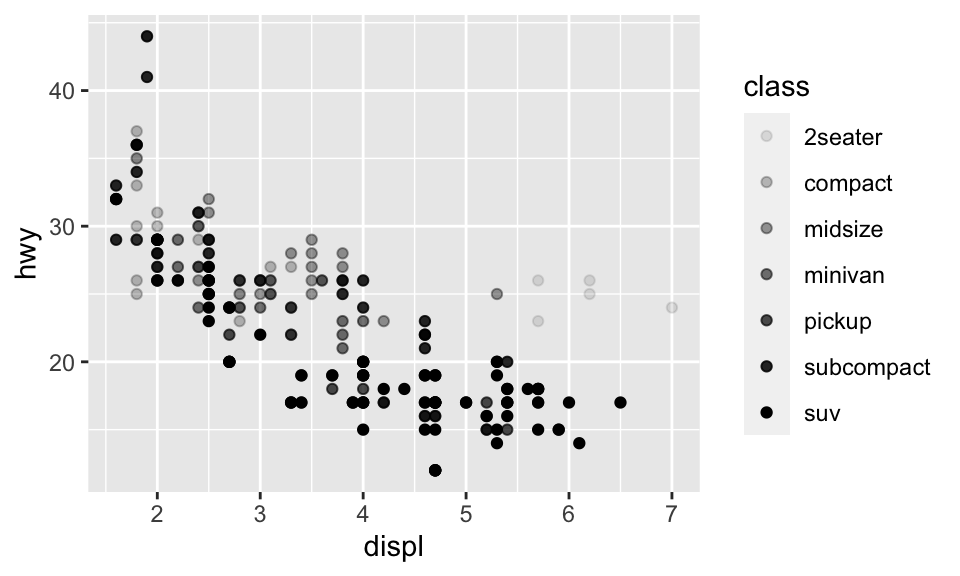ggplot(data = mpg) + geom_point(mapping = aes(x = displ, y = hwy, shape = class))
## Warning: The shape palette can deal with a maximum of 6 discrete values because
## more than 6 becomes difficult to discriminate; you have 7. Consider
## specifying shapes manually if you must have them.
## Warning: Removed 62 rows containing missing values (geom_point).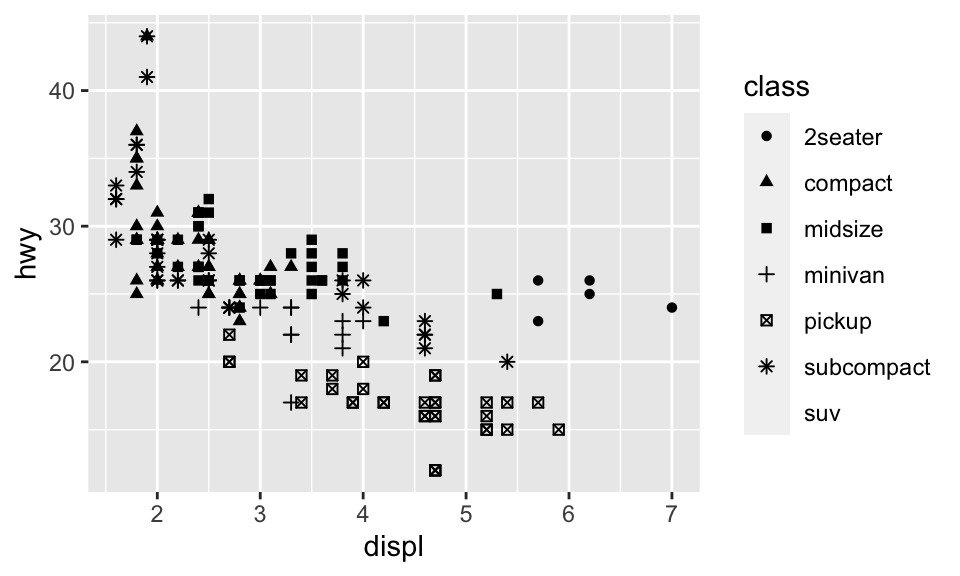ggplot(data = mpg) + geom_point(mapping = aes(x = displ, y = hwy), color = "blue")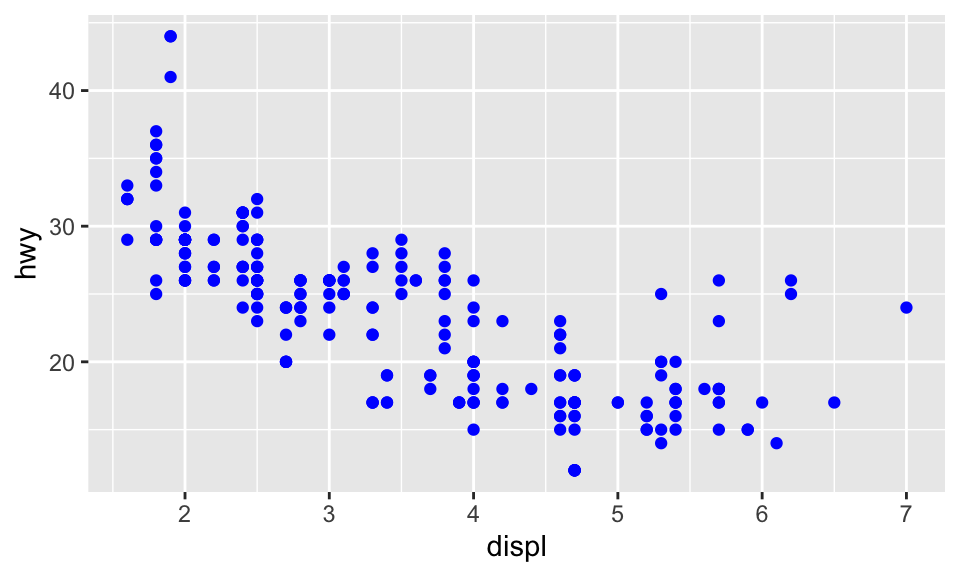## 5.4 Facets

ggplot(data = mpg) +
geom_point(mapping = aes(x = displ, y = hwy)) +
facet_wrap(~ class, nrow = 2)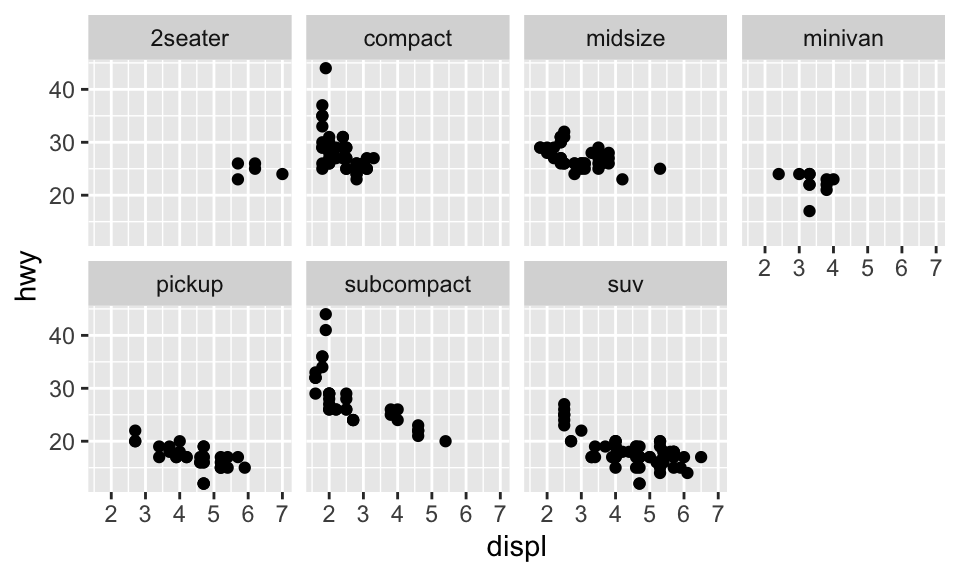ggplot(data = mpg) +
geom_point(mapping = aes(x = displ, y = hwy)) +
facet_grid(drv ~ cyl)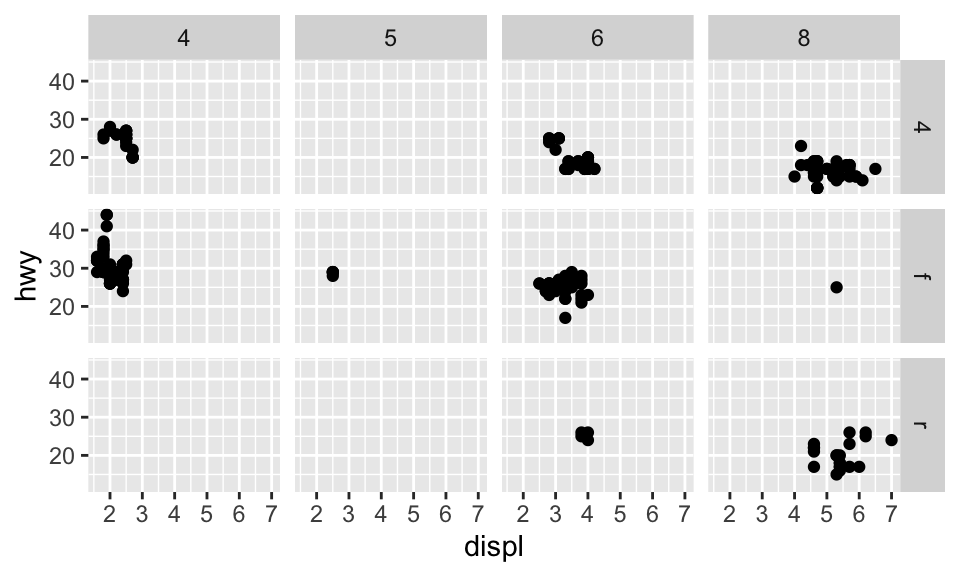## 5.5 幾何物件

Geom 是一種圖用來表示資料的幾何物件。例如，bar charts 使用 bar geoms，line charts 使用 line geoms，boxplots 使用 boxplot geoms 等。

# 對於 geom_smooth() 中的 method 與 formula 用法可見其文檔
ggplot(data = mpg) +
geom_smooth(mapping = aes(x = displ, y = hwy), method = 'loess', formula = 'y ~ x')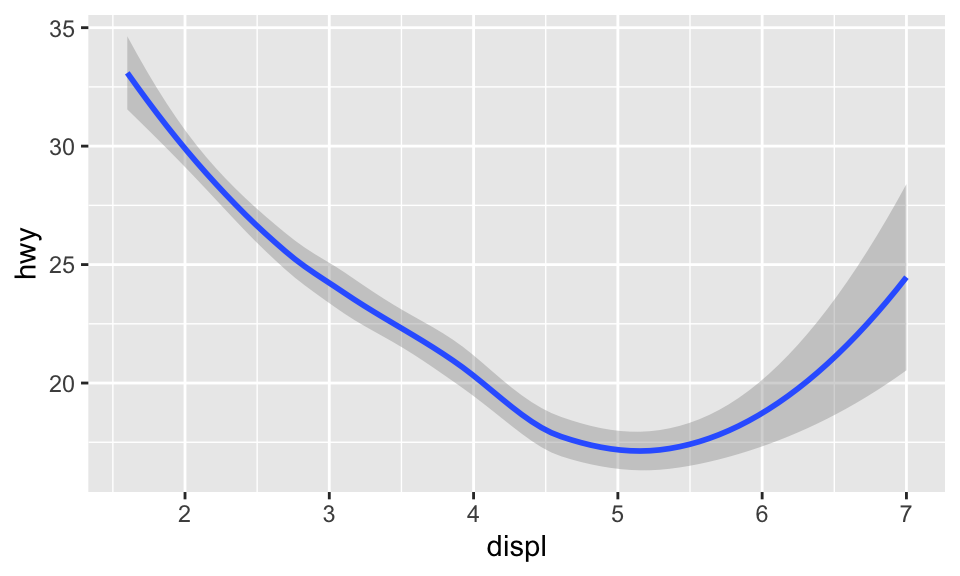ggplot(data = mpg) +
geom_smooth(mapping = aes(x = displ, y = hwy, linetype = drv),
method = "loess", formula = "y ~ x")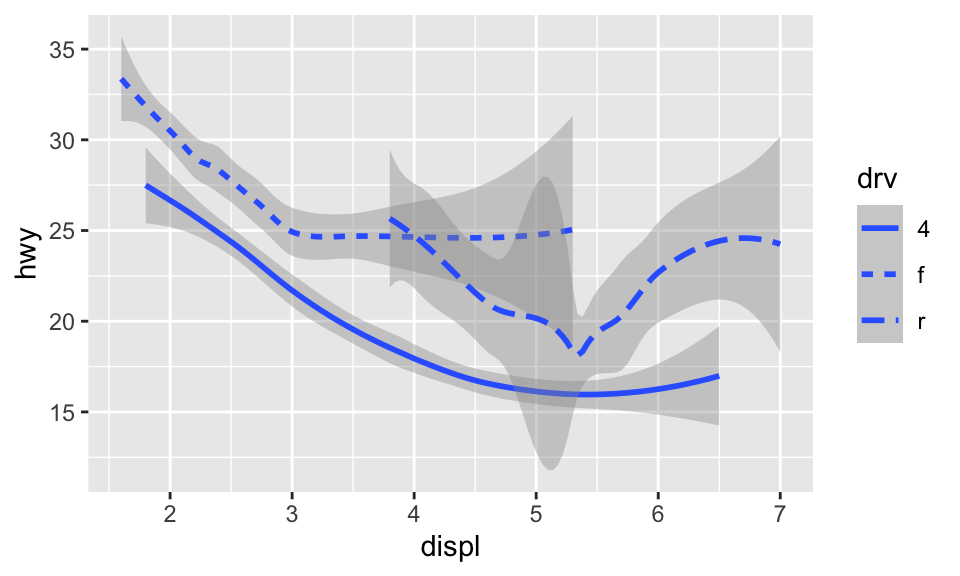ggplot(data = mpg) +
geom_smooth(mapping = aes(x = displ, y = hwy, color = drv, linetype = drv),
method = "loess", formula = "y ~ x") +
geom_point(mapping = aes(x = displ, y = hwy, color = drv))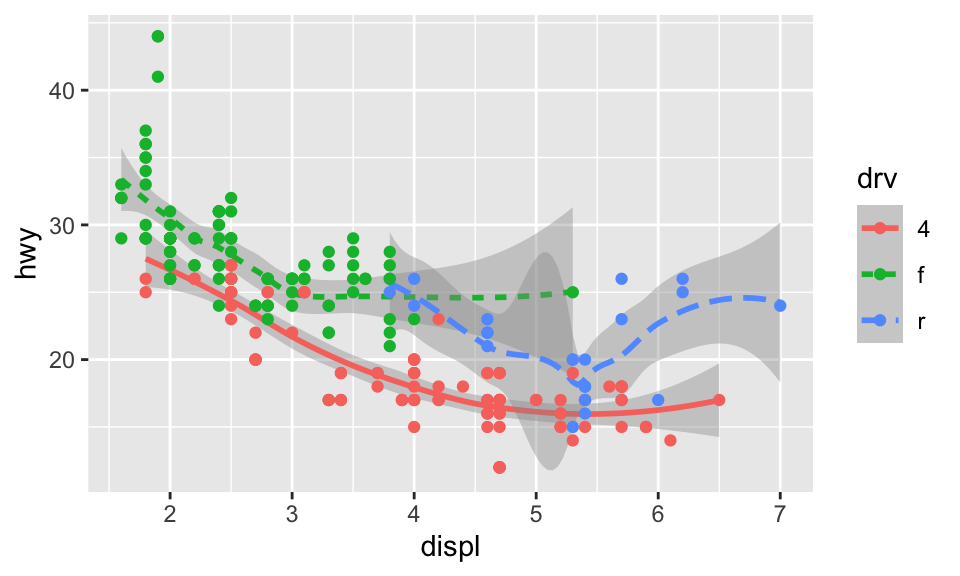ggplot(data = mpg, mapping = aes(x = displ, y = hwy, color = drv)) +
geom_smooth(aes(linetype = drv), method = "loess", formula = "y ~ x") +
geom_point()## 5.6 統計轉換

diamondsggplot2 中的一個 dataset，約有 54000 顆鑽石的資料，包含 caratcutcolorclaritydepthtableprice 等變數。使用 geom_bar() 可以依據某個變數畫出長條圖（bar chart），例如我們想要知道各種 cuts 到底有分別有多少鑽石，可以：

ggplot(data = diamonds) + geom_bar(mapping = aes(x = cut))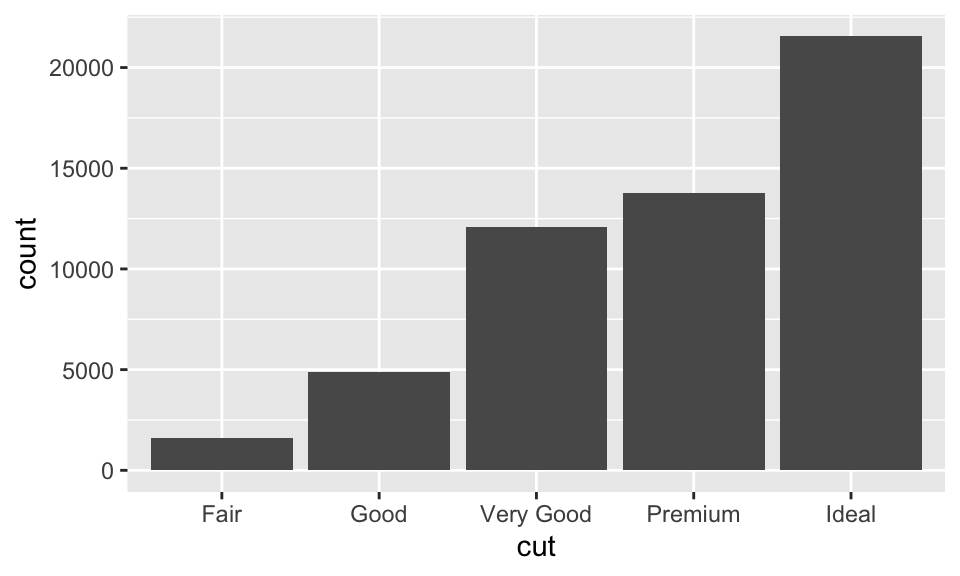1. 長條圖、直方圖（histogram）或 frequency polygons 都會計算個數。
2. Smoothers 會適配模型然後畫出預測。
3. Boxplots 會計算分佈。

1. 想要替換預設的 stat 的時候。

2. 想要換原本的 mapping 時。

ggplot(data = diamonds) +
geom_bar(
mapping = aes(x = cut, y = ..prop.., group = 1)
)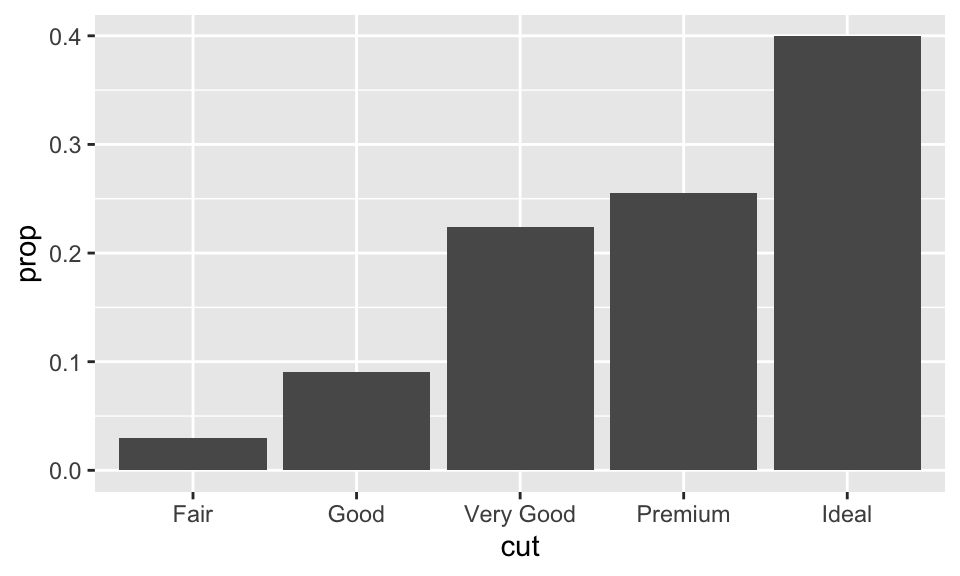1. 想要使用其他的 statistical transformation 的時候。例如使用 stat_summary()，其會對每個 x 都 summarizes 其 y
ggplot(data = diamonds) +
stat_summary(
mapping = aes(x = cut, y = depth),
fun.min = min,
fun.max = max,
fun = median
)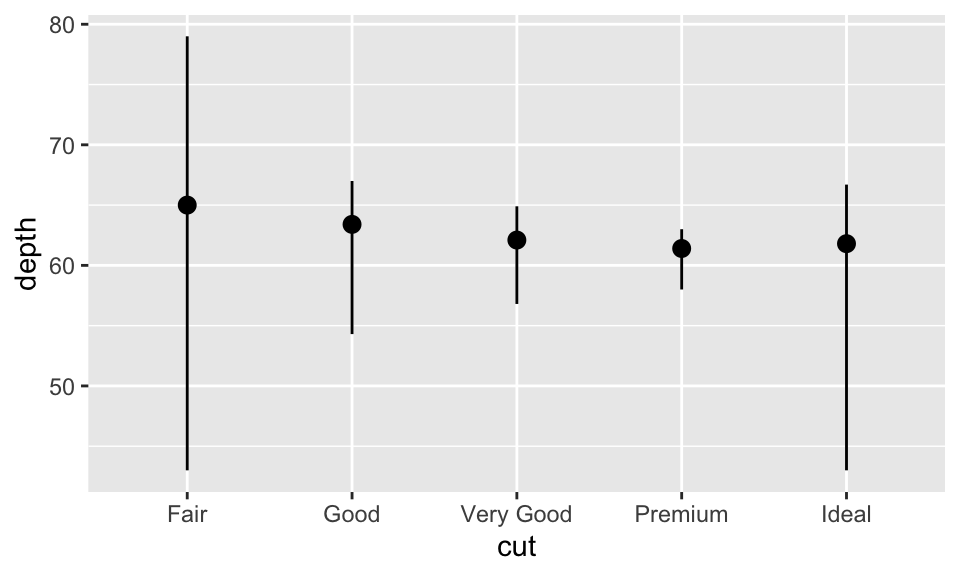ggplot(data = diamonds) +
geom_bar(mapping = aes(x = cut, color = cut))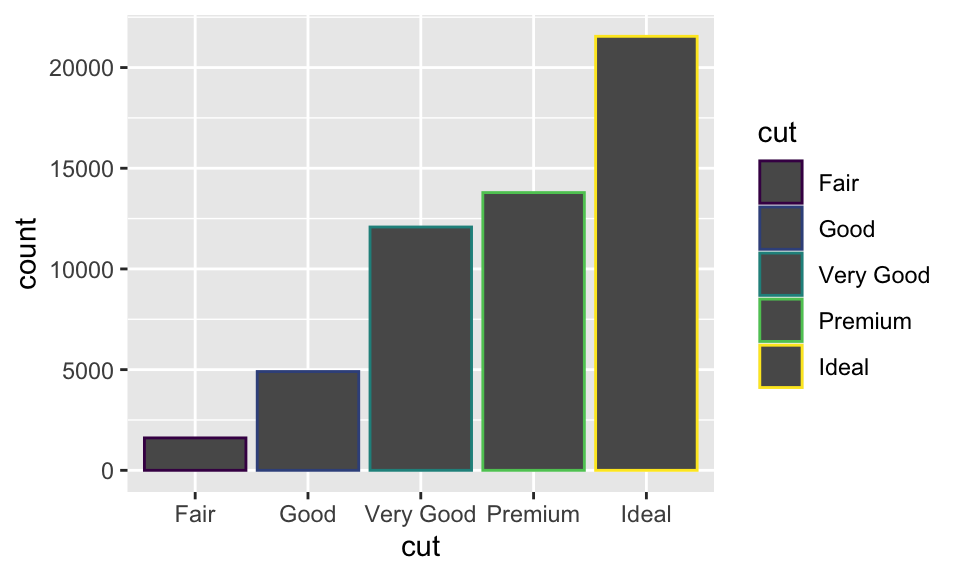ggplot(data = diamonds) +
geom_bar(mapping = aes(x = cut, fill = cut))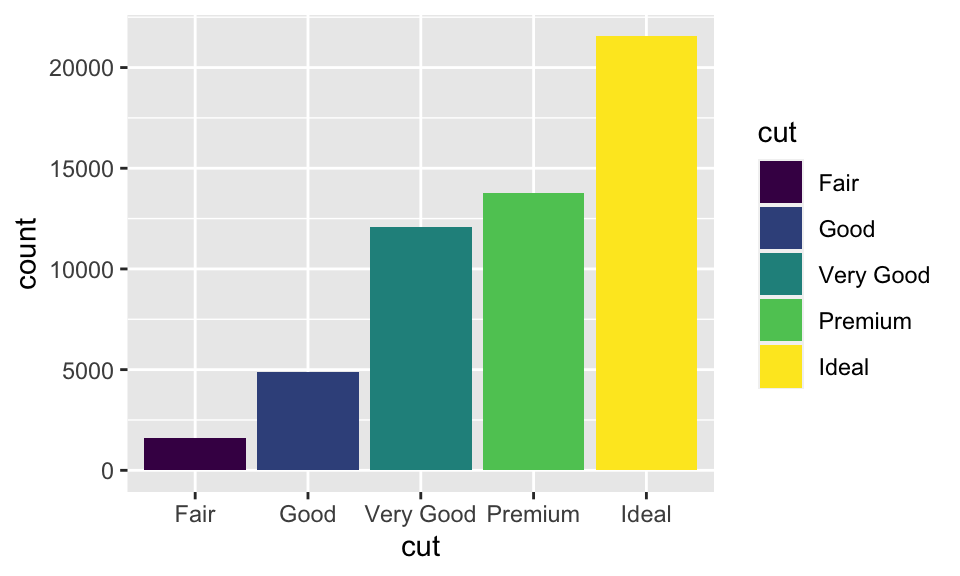ggplot(data = diamonds, mapping = aes(x = cut, fill = clarity)) +
geom_bar()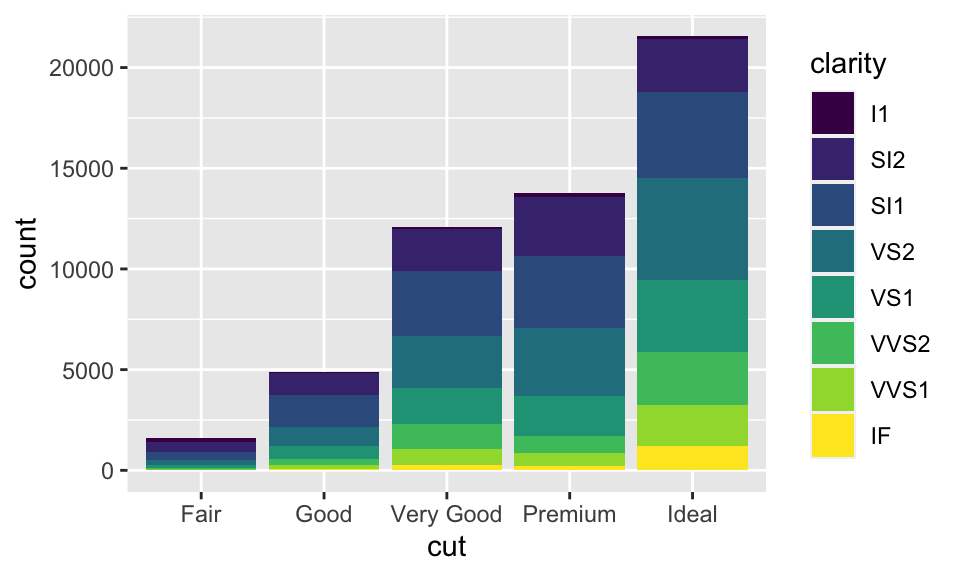1. position = "identity"：用在 bar chart 上效果有點像 stack，但差別在調整透明度後可以看出來（即 alpha = 1/5）；雖然還是不明顯，但差別在 identity 的各個物件是會相互堆疊的。例如在下圖中，調整透明度明明應該所有物件的透明度都相同，但越靠下的部分透明度顯然越低，這就是因為越靠下的部分有越多個物件重疊在一起，使得圖形顯得較不透明。
ggplot(data = diamonds, mapping = aes(x = cut, fill = clarity)) +
geom_bar(alpha = 1/5, position = "identity")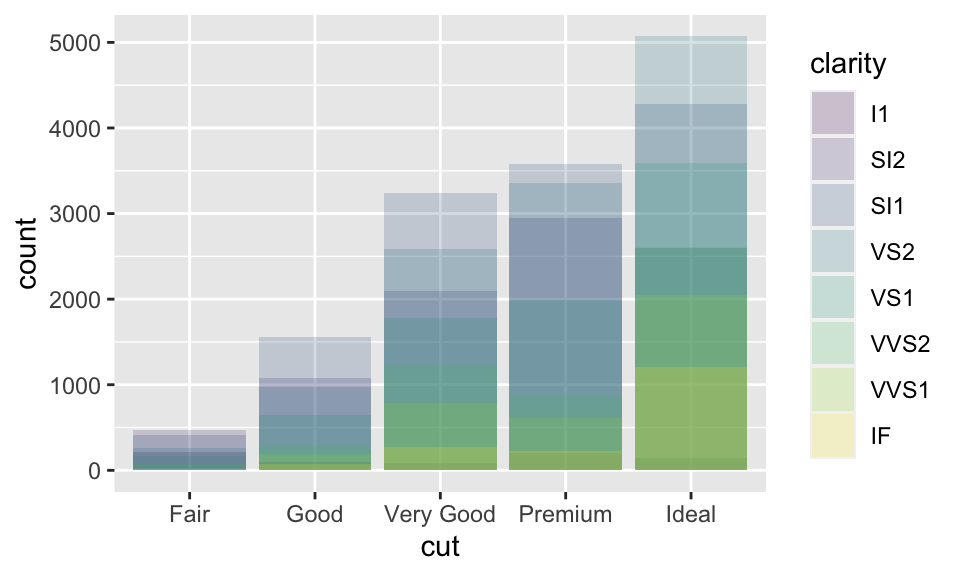1. position = "fill"：依據指定的變數（此數為 clarity）堆疊，差別在高度相同，所以便於我們比較組間的比例差別。
ggplot(data = diamonds, mapping = aes(x = cut, fill = clarity)) +
geom_bar(position = "fill")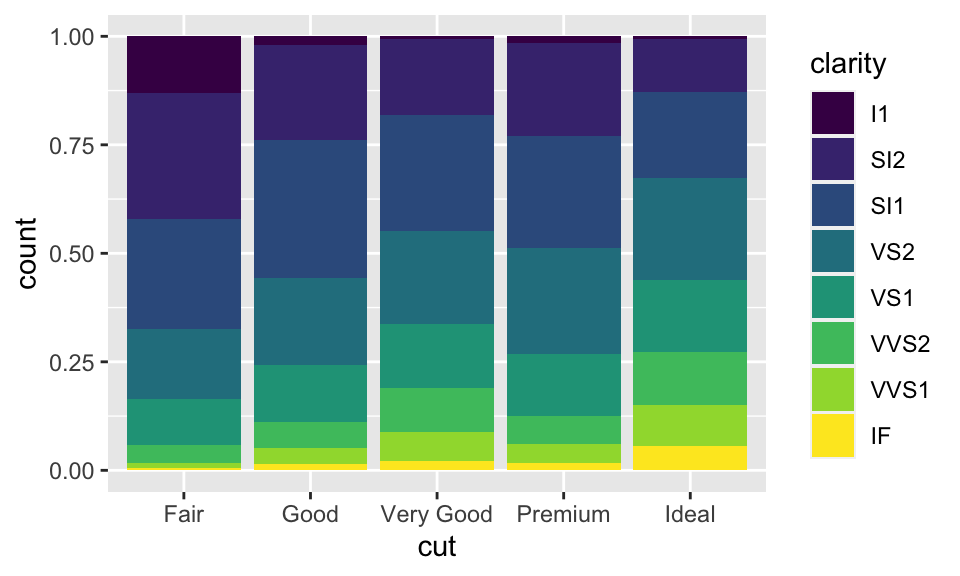1. position = "dodge"：以此例而言，即是對於每個不同的 cut，都分別把其各個 clarity 展現在逐一呈現，而非用堆疊的方式。
ggplot(data = diamonds, mapping = aes(x = cut, fill = clarity)) +
geom_bar(position = "dodge")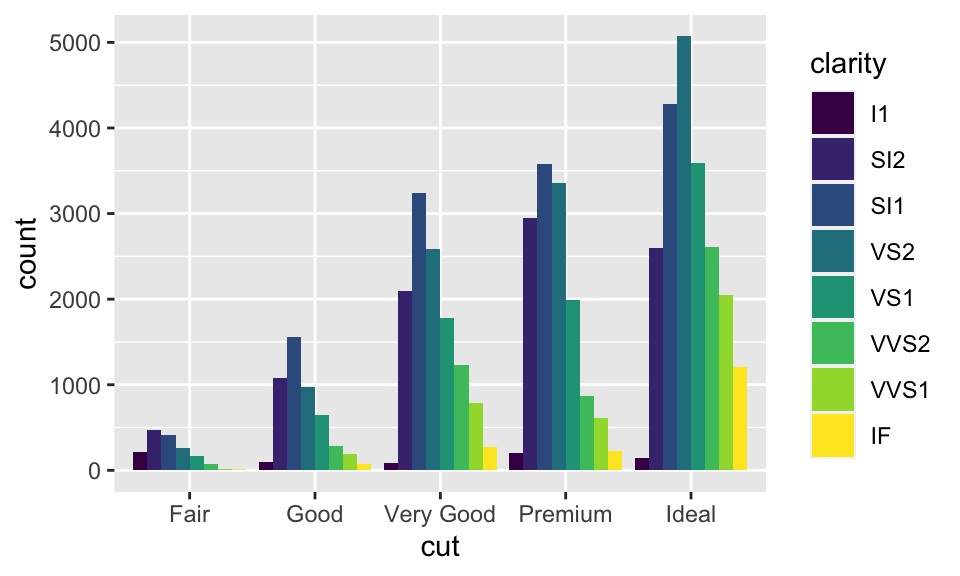ggplot(data = mpg) + geom_point(mapping = aes(x = displ, y = hwy))str(mpg)
# tibble [234 × 11] (S3: tbl_df/tbl/data.frame)
# ...

ggplot(data = mpg, mapping = aes(x = displ, y = hwy)) +
geom_point(position = "jitter")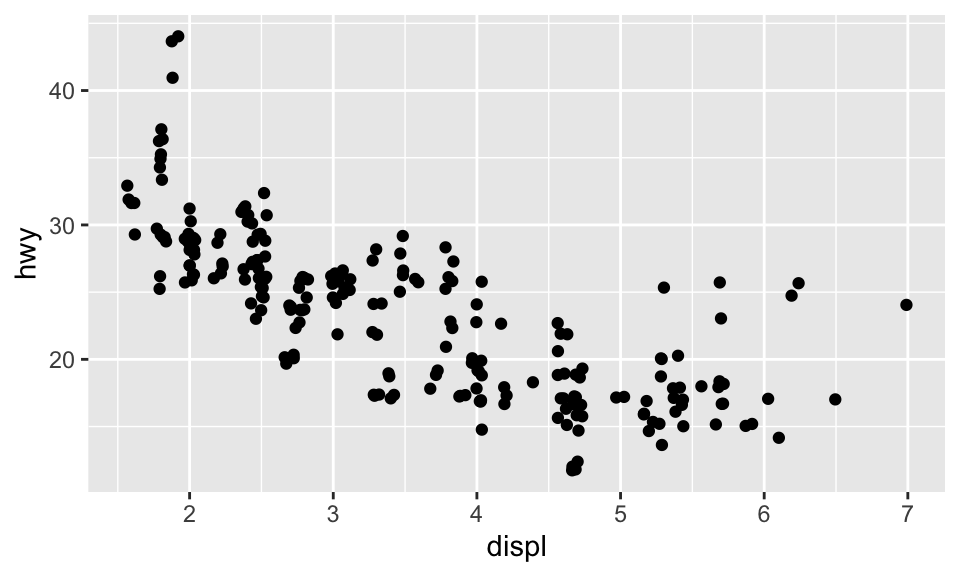## 5.8 座標系統

1. coord_flip()：繪製旋轉 90 度的直角坐標，如：
ggplot(data = mpg, mapping = aes(x = class, y = hwy)) + geom_boxplot()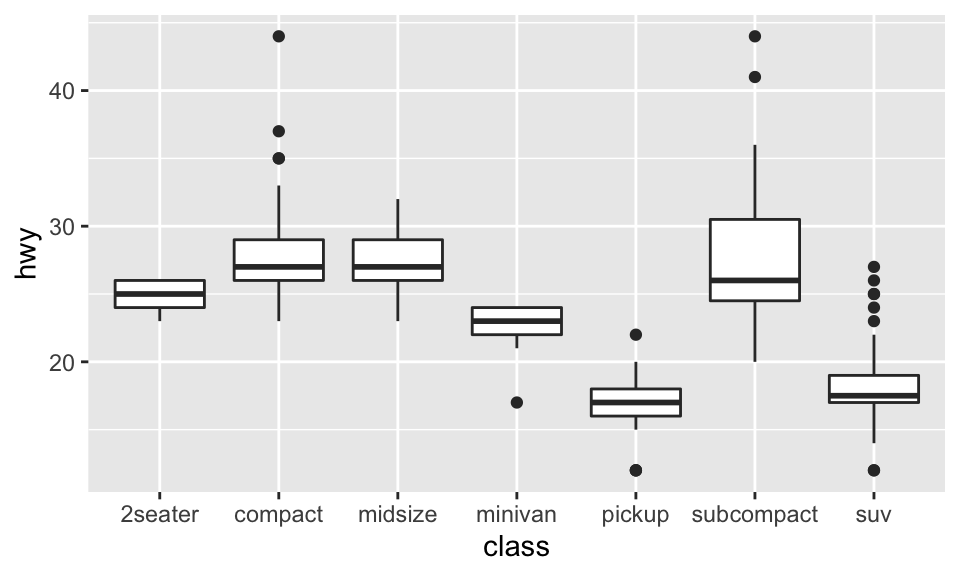ggplot(data = mpg, mapping = aes(x = class, y = hwy)) + geom_boxplot() + coord_flip()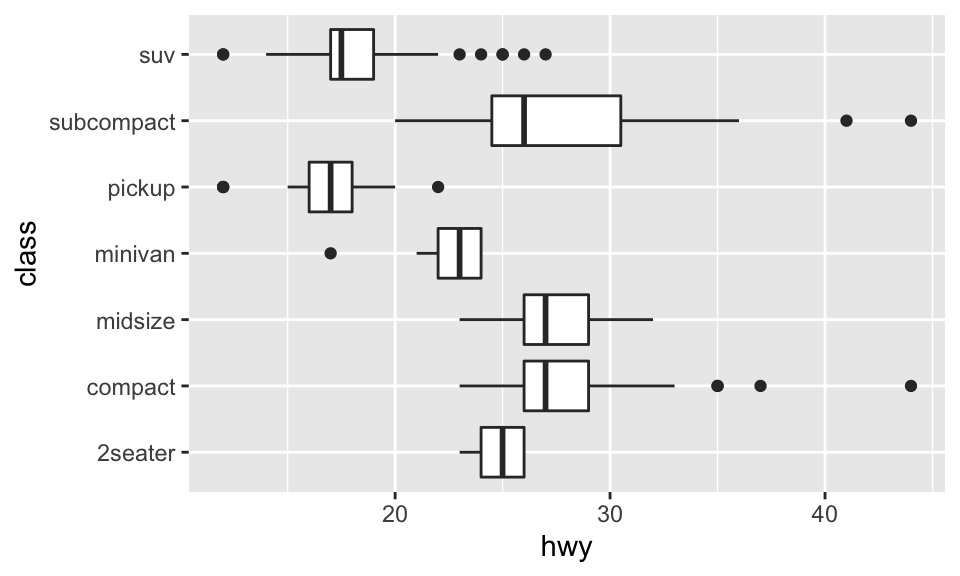1. coord_quickmap()：繪製有正確方位比例的地圖4，如：
nz <- map_data("nz")

ggplot(nz, aes(long, lat, group = group)) +
geom_polygon(fill = "white", color = "black")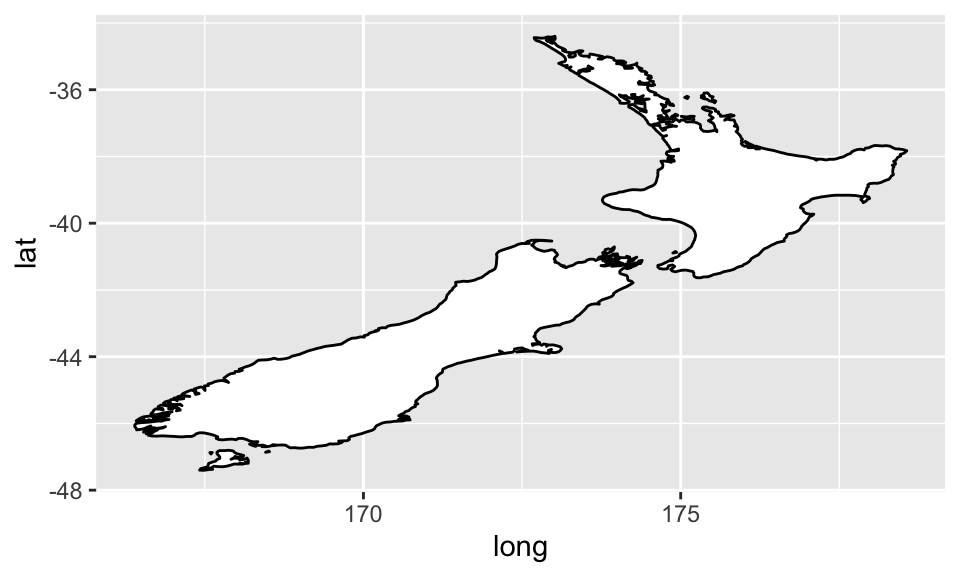ggplot(nz, aes(long, lat, group = group)) +
geom_polygon(fill = "white", color = "black") + coord_quickmap()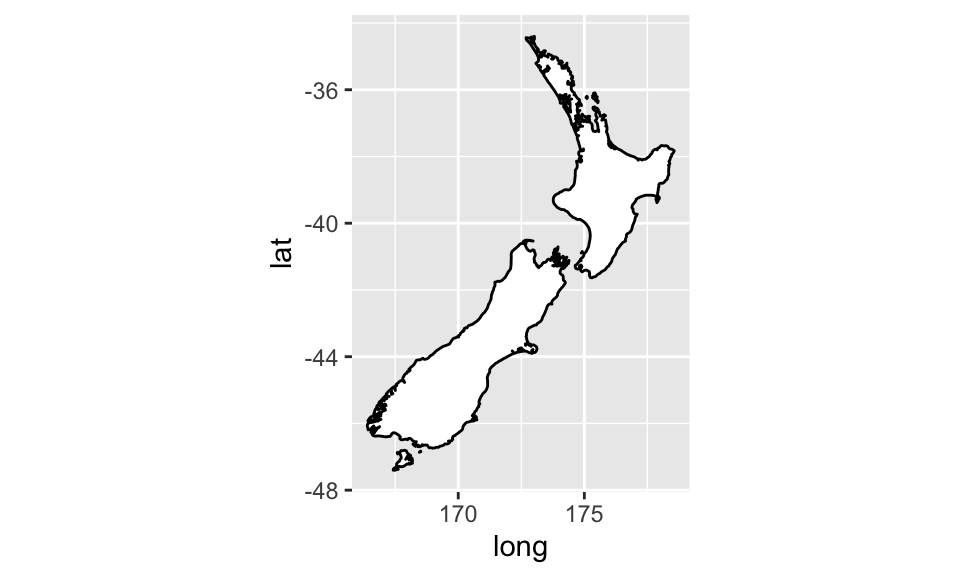1. coord_polar()：繪製極座標圖（polar coordinates），結合 bar char 與 Coxcomb chart，如：
bar <- ggplot(data = diamonds) +
geom_bar(mapping = aes(x = cut, fill = cut), show.legend = FALSE, width = 1) +
theme(aspect.ratio = 1) + labs(x = NULL, y = NULL)

bar + coord_polar()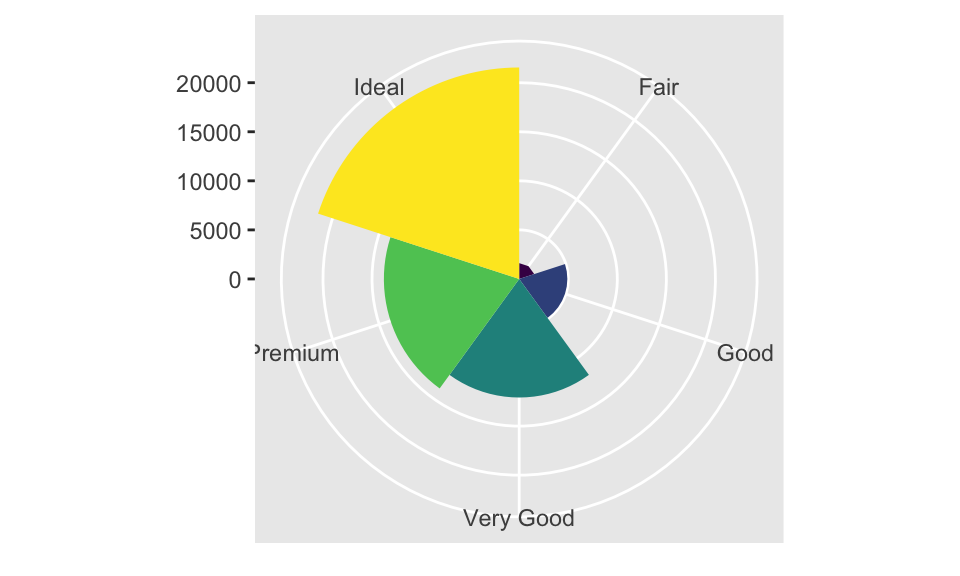1. coord_fixed：因為人對與 45 度線的差異感受最明顯，而 coord_fixed() 可以讓 geom_abline()（用以產生 reference lines 的 geom）產生的線為 45 度，如：
p <- ggplot(data = mpg, mapping = aes(x = cty, y = hwy)) +
geom_point() + geom_abline()

p + coord_fixed()  # 如果不加 coord_fixed()，比例將會跑掉。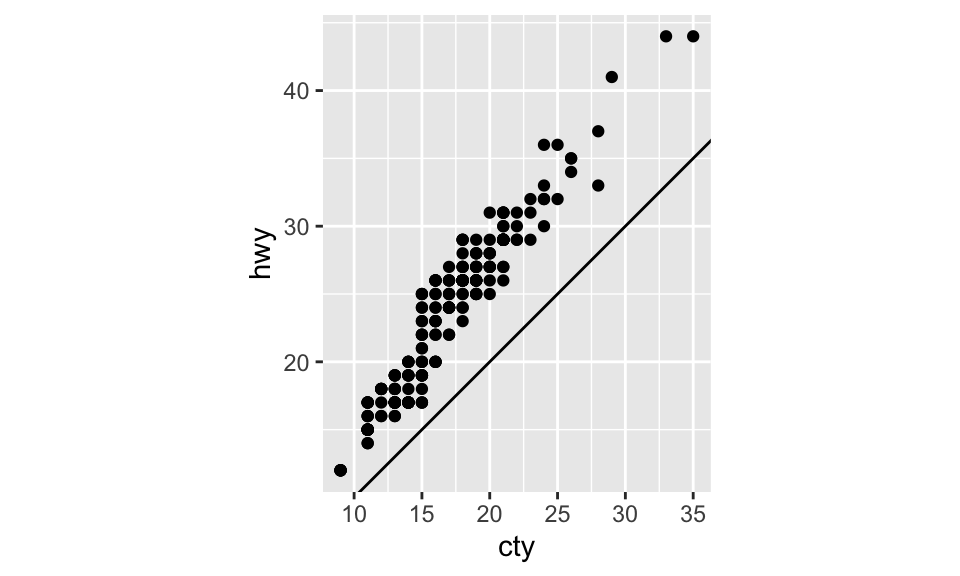## 5.9 標籤

labs() 能使我們為圖加上標題、註解或更改 x 軸、y 軸的名稱，如：

ggplot(data = mpg, mapping = aes(x = class, y = hwy)) +
geom_boxplot() +
coord_flip() +
labs(y = "Highway MPG",
x = "Class",
title = "Highway MPG by car class",
subtitle = "1999-2008",
caption = "Source: http://fueleconomy.gov")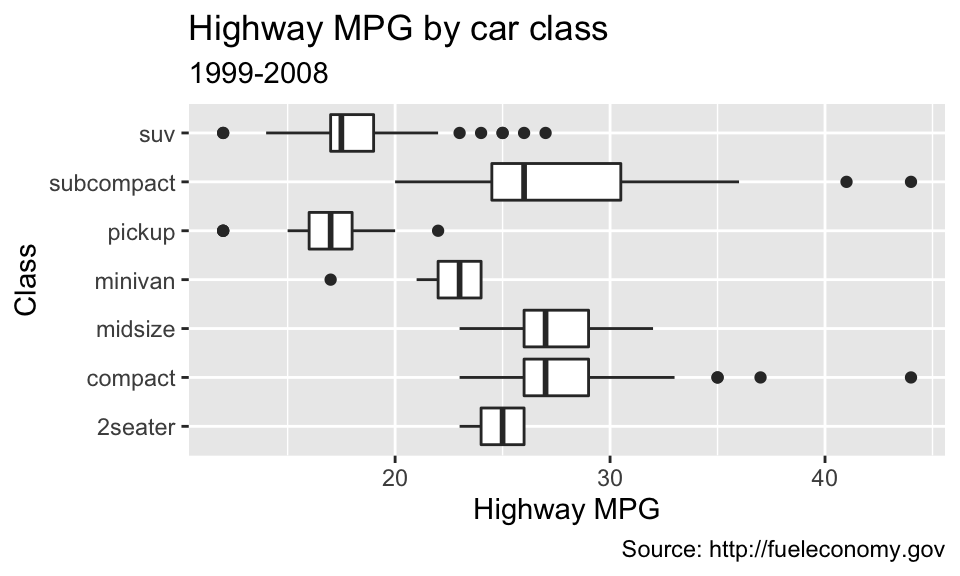### 參考文獻

Wickham, Hadley, and Garrett Grolemund. 2016. R for Data Science. First Edition. O’Reilly Media, Inc.

1. 另有 coord_map()，功能與 coord_quickmap() 類似。coord_map() 預設使用麥卡托投影法（Mercator projection）。而兩者的差別在 coord_quickmapcoord_map() 的近似，忽略了地球在不同經緯度比例的曲度差異，因此運行速率較快，但也稍微不準確。↩︎